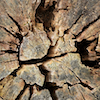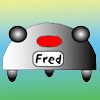#### You may also like### Transformations on a Pegboard

How would you move the bands on the pegboard to alter these shapes?### Timber!

How can the school caretaker be sure that the tree would miss the school buildings if it fell?### Fred the Class Robot

Billy's class had a robot called Fred who could draw with chalk held underneath him. What shapes did the pupils make Fred draw?

# Triangle Pin-down

##### Age 7 to 11Challenge Level
A lot of students interpreted the "same type of triangle" as a right-angled triangle that is also isosceles. Emma, aged 13 wrote : To get an exact copy of the right-angled triangle you need the number of points to be a multiple of 4.

If you interpret "same type of triangle" to mean any right-angled triangle, your answer would be different. What would it be?

Bob has an answer for the six-pin board :

For the equilateral triangle the number of pegs must be a multiple of 3.

Can you give an explanation for these observations?

Thank you to Kyle from New Zealand who correctly identified certain circles and states the type of triangle as

Isosceles.

If you consider that an isosceles triangle can also be a right-angled triangle, then many answers are possible. Do you think that we can consider an equilateral triangle to be a special form of isosceles triangle?

If you consider that some triangles can be more than one type of triangle at the same time, can you work out the answer to the nine-pin part of the question?